# How many types of acceleration are there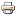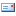This kinematics script is intended to describe the fundamentals of movement and what is meant by this in the field of physics. The different types of movement are also presented here and important key terms such as the space curve or the mass point are explained.

### Move

The change in position of a body in relation to a body at rest or to the position of the observer corresponds to a movement in the sense of physics.

### Space curve in the coordinate system

There are many forms of motion, such as circular motion and rectilinear motion. Accordingly, a correspondingly suitable coordinate system is selected for the physical description of the respective movement. The observer assumed to be at rest is mostly at the origin of the coordinate system, while the observed body runs through a three-, two- or one-dimensional space curve (trajectory) with respect to this origin.

### Mass point / point mass

In many cases, the physical description of a movement becomes easier for you if you imagine the observed body as a single point in the corresponding coordinate system. This point is treated as if it had the entire mass of the body. The center of gravity of the body is selected as a so-called "mass point" (often also as a "point mass").

Two fields of physics deal with motion:

Kinematics is the study of the description of movements. In technical mechanics, dynamics represent kinetics, which is a study of the causes of movements. The kinetics are in contrast to the statics, which only deal with systems at rest.

### The relativity of motion

The description of a movement of an observed object always depends on the observer. For example, a person in the passenger seat of a moving car appears to be moving from the perspective of a passer-by on the edge of the road, while from the perspective of the motorist he appears to be at rest.

### The rectilinear uniform movement:

A straight, uniform movement exists when the trajectory represents a straight line section, while the speed is the same at every point along the trajectory and the acceleration is zero everywhere.### Evenly accelerated movement:

In the case of a uniformly accelerated movement, the direction and the amount of acceleration are the same at every point on the trajectory. The trajectory can be a straight line or a parabola.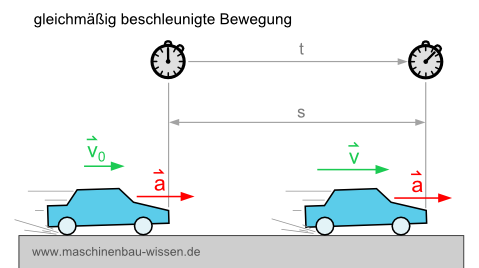### Periodic movement:

If the observation object returns to the starting point after a certain time (period) and has the same speed and direction, then it is a periodic movement. Such movements always have closed trajectories. A special case of periodic motion is, for example, circular motion.

### Circular motion:

The circular movement forms a circular trajectory. The speed vector forms a right angle with the radius at every point in time. Therefore, this vector has a tangential direction. It is always a uniform circular movement if the amount of the speed is constant throughout the circular movement. With uniform circular motion, the normal acceleration is always directed towards the center of the circle and the tangential acceleration is zero.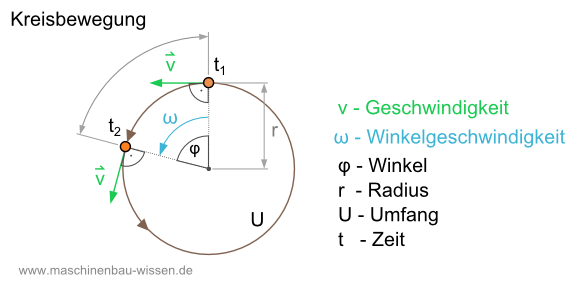### Harmonic oscillation:

The harmonic oscillation is also a periodic movement. The change in place over time always follows a sine function in the case of a periodic movement.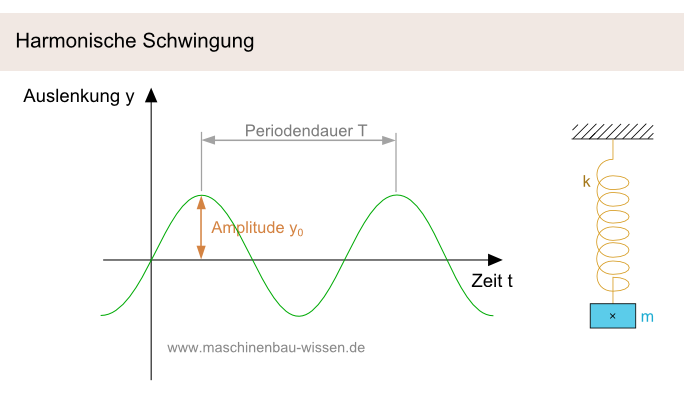Any object that is slightly deflected from its equilibrium position generally vibrates harmoniously. Every periodic movement can be represented as the sum of harmonic oscillations using Fourier analysis. The frequencies of these individual oscillations are multiples of the basic frequency. This frequency is the reciprocal of the period duration. A classic example is the spring pendulum, with which, among other things, harmonic oscillations are described and illustrated in physics and kinetics.